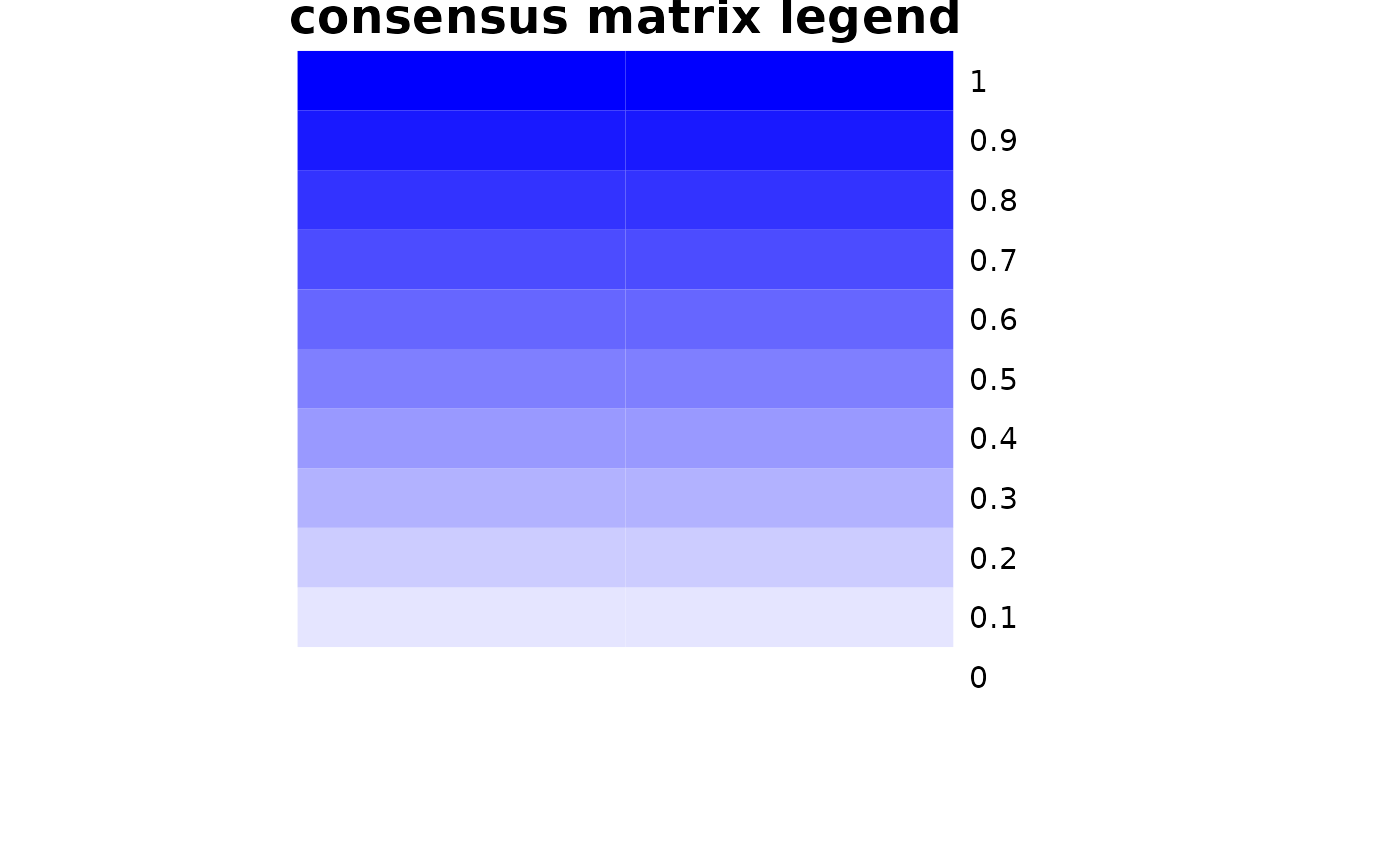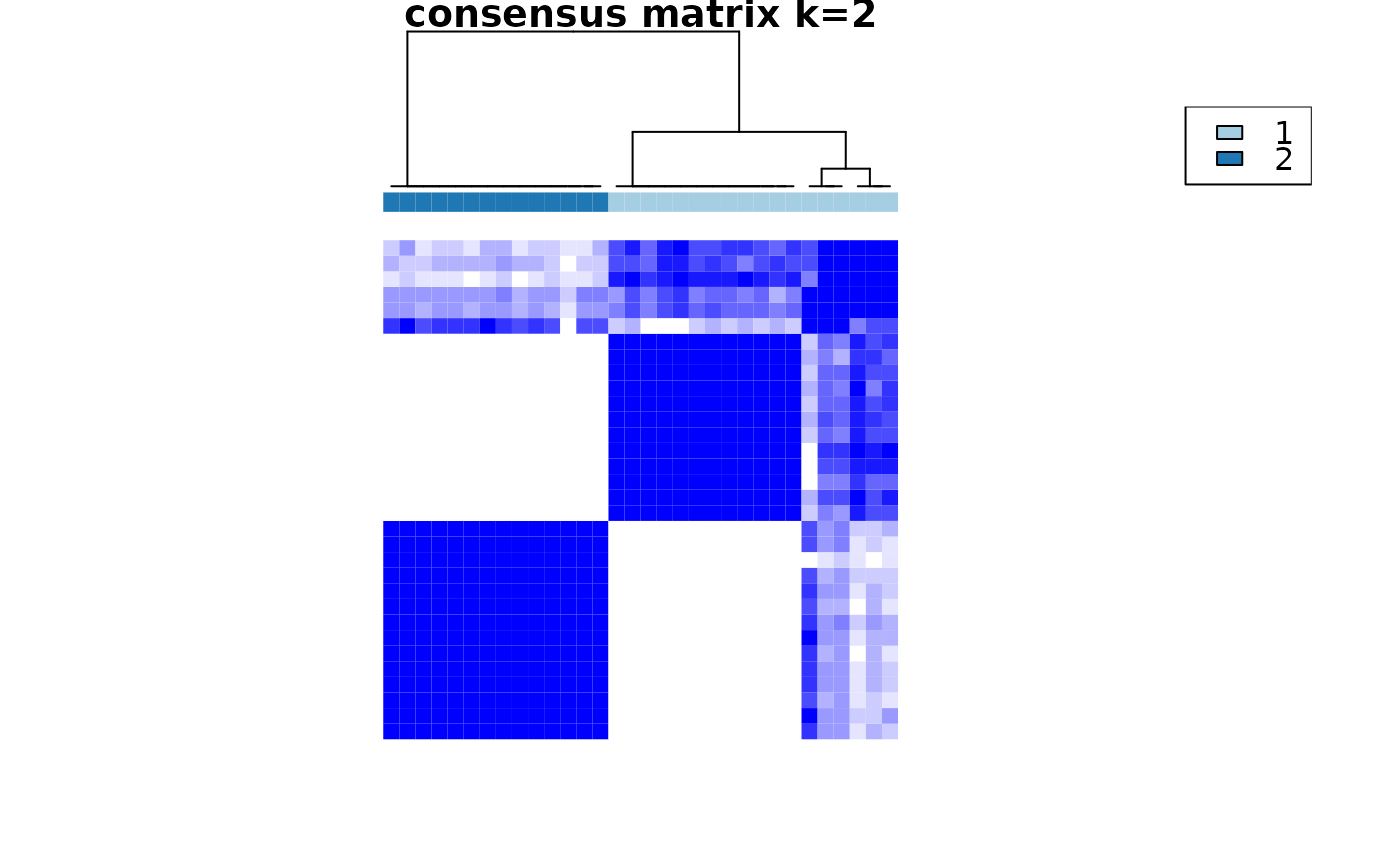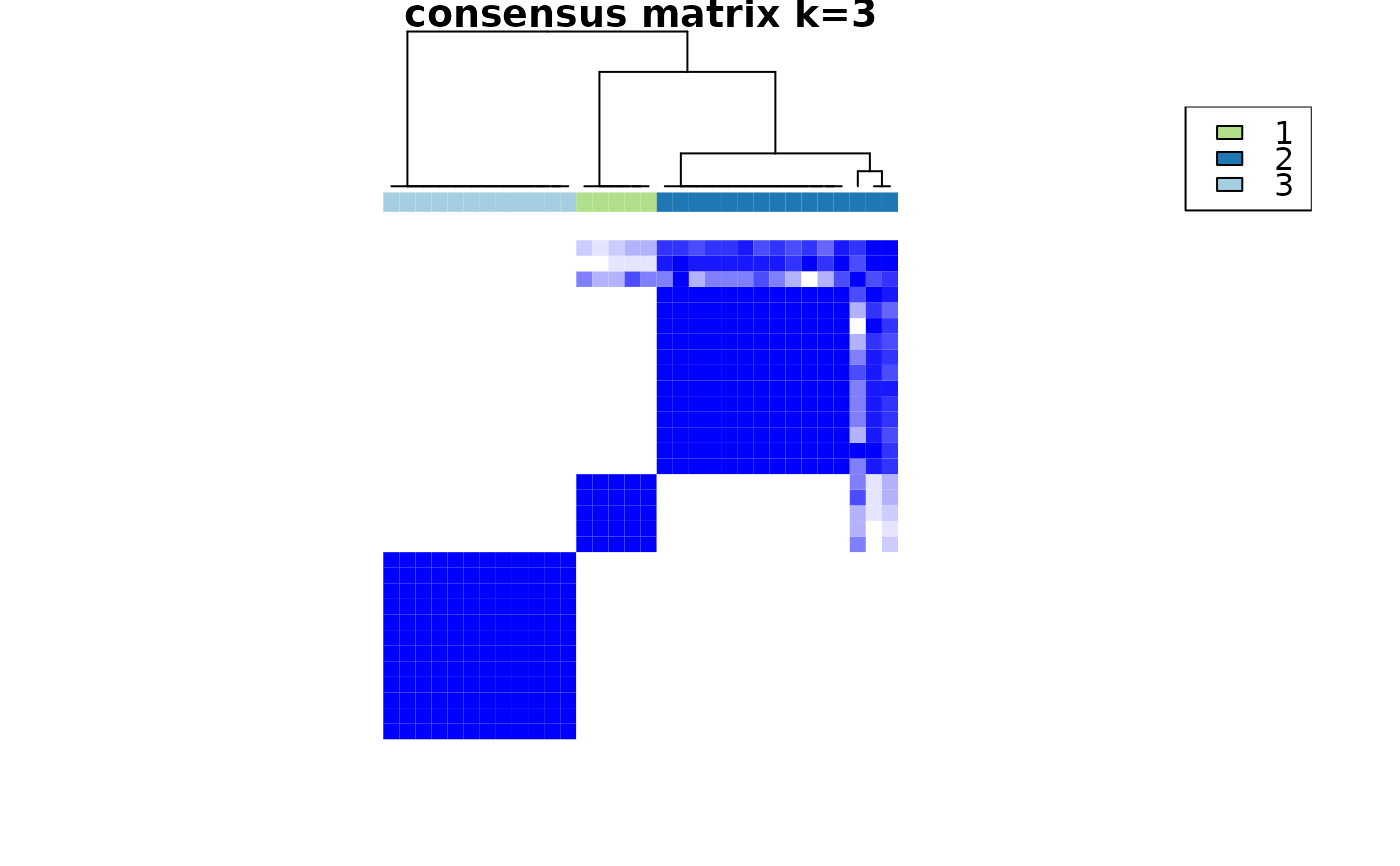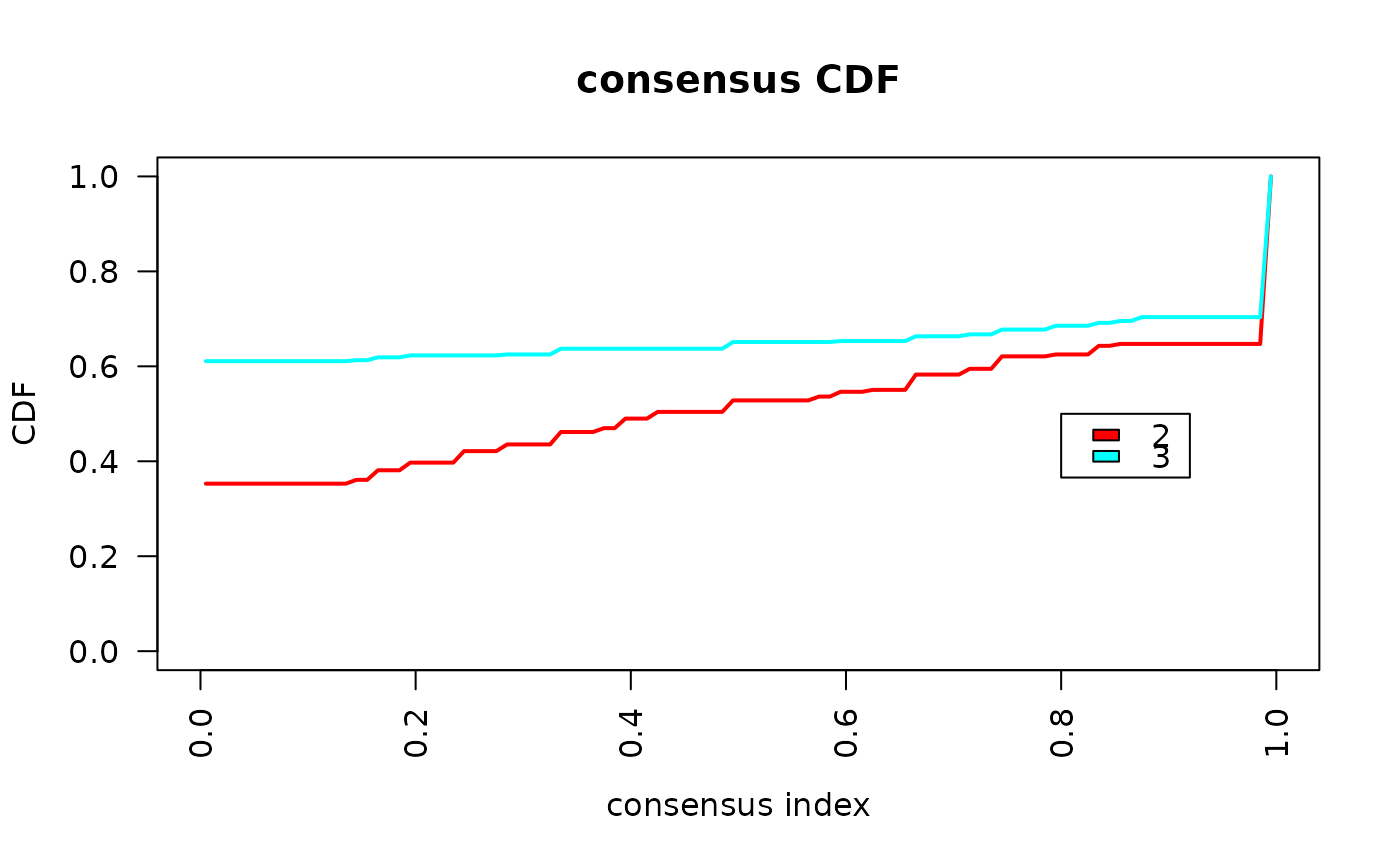This function uses the ConsensusClusterPlus function from the package with the same name with defaults for clustering data with categorical variables. As the distance function, the Gower distance is used.

## Usage

crosssectional_consensus_cluster(
data,
reps = 1000,
...
)

## Arguments

data

a matrix or data.frame containing variables that should be used for computing the distance. This argument is passed to StatMatch::gower.dist

reps

number of repetitions, same as in ConsensusClusterPlus

linkage method for final clustering, same as in ConsensusClusterPlussame as in ConsensusClusterPlus

linkage method for clustering steps, same as in ConsensusClusterPlus

...

other arguments passed to ConsensusClusterPlus, attention: the d argument can not be set as it is directly computed by crosssectional_consensus_cluster

## Value

The output is produced by ConsensusClusterPlus

## Details

data can take all input data types that gower.dist can handle, i.e. numeric, character/factor, ordered and logical.

## Examples

dc <- mtcars
# scale continuous variables
dc <- sapply(mtcars[, 1:7], scale)
# code factor variables
dc <- cbind(as.data.frame(dc),
vs = as.factor(mtcars$vs), am = as.factor(mtcars$am),
gear = as.factor(mtcars$gear), carb = as.factor(mtcars$carb))
cc <- crosssectional_consensus_cluster(
data = dc,
reps = 10,
seed = 1
)
#> end fraction
#> clustered#> clustered